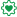# QlikView Layout & Visualizations

Discussion Board for collaboration on QlikView Layout & Visualizations.

Announcements
Modernize Your QlikView Deployment webinar, Nov. 3rd. REGISTER
cancel
Showing results for
Did you mean:
HighlightedNot applicable

## Expression doesn't look at date

Hi,

In a text box I have the below expression:

=Interval(

(RangeMin(frac(timestamp(vJD_TIME, MakeTime(18)))))

- RangeMax(frac(logdate_true), MakeTime(8))

//+ (NetWorkDays(logdate_true, vJD_TIME) * MakeTime(0)) // Only 10 hours per whole day

)

In my Load Script I have set the following variable LET vJD_TIME = now()

In my text box I am trying to calcuate the difference between the current system time (variable: vJD_TIME) minus the time the call was logged (field logdate).

This works correctly when logdate is the same date as the current system time, however when the logdate is another date ie: 16/12/2012 16:30:00, it doesnt take the date into account and sometimes shows a minus figure.

How can I correct this?

Regards,

Jon Ditchfield

1 Solution

Accepted Solutions
HighlightedEmployee

Hi Jon,

It does. So something like this to take into account the number of days between now and the logdate, given that every day should count as 10 net work hours and not 24?

`=Interval(Fabs(Interval(          RangeMin(frac(timestamp(vJD_TIME)), MakeTime(18))          - RangeMax(frac(timestamp('16/12/2012 16:30:00')), MakeTime(8))          + (Interval#(10, 'hh') * Interval(Day(timestamp(vJD_TIME)) - Day(timestamp('16/12/2012 16:30:00')))) )))`

That should return roughly speaking 2 hours (14:30 -16:30) plus (10h times 2 days of 10 hours) = 18 hours

or

16/12/2012 16:30 - 18:00 = 1,5h

+ 17/12/2012 (whole day) = 10h

+ 18/12/2012 08:00 - 14:30 = 6,5h

Total 18h

Hope that helps.

Miguel

4 Replies
HighlightedEmployee

Hi Jon,

The same expression (some parentheses are missing, probably a typo when pasting the code) is working for me, and it does return a negative value, but for me that makes sense. I mean, it's 13:30, so the RangeMin() with 18:00 will return 13:30.

The RangeMax() between 16:30 according to your example, and 08:00 will return 16:30. So 13:30 - 18:30 = -03:00 (negative)

If that logic is correct for what you are looking for, wrap the whole expression with Fabs() to get the absolute value and Interval() to make it look like hh:mm:ss:

`=Interval(Fabs(Interval(          RangeMin(frac(timestamp(vJD_TIME)), MakeTime(18))          - RangeMax(frac(timestamp('16/12/2012 16:30:00')), MakeTime(8)))))`

Hope that helps.

Miguel

HighlightedNot applicable

Hi Miguel,

Thanks for the script, however on some of the records the logdate field could be for example yesterday, therefore say the logdate is 17/12/2012 08:00:00 and the vJD_TIME is 18/12/2012 09:00:00, instead of it showing 01:00:00, we would want it to show 11:00:00, as it has been 11 hours on the backlog.  Hope this makes sense?

Regards,

Jon

HighlightedEmployee

Hi Jon,

It does. So something like this to take into account the number of days between now and the logdate, given that every day should count as 10 net work hours and not 24?

`=Interval(Fabs(Interval(          RangeMin(frac(timestamp(vJD_TIME)), MakeTime(18))          - RangeMax(frac(timestamp('16/12/2012 16:30:00')), MakeTime(8))          + (Interval#(10, 'hh') * Interval(Day(timestamp(vJD_TIME)) - Day(timestamp('16/12/2012 16:30:00')))) )))`

That should return roughly speaking 2 hours (14:30 -16:30) plus (10h times 2 days of 10 hours) = 18 hours

or

16/12/2012 16:30 - 18:00 = 1,5h

+ 17/12/2012 (whole day) = 10h

+ 18/12/2012 08:00 - 14:30 = 6,5h

Total 18h

Hope that helps.

Miguel

HighlightedNot applicable

Thanks for your help Miguel, it works how I want it to.